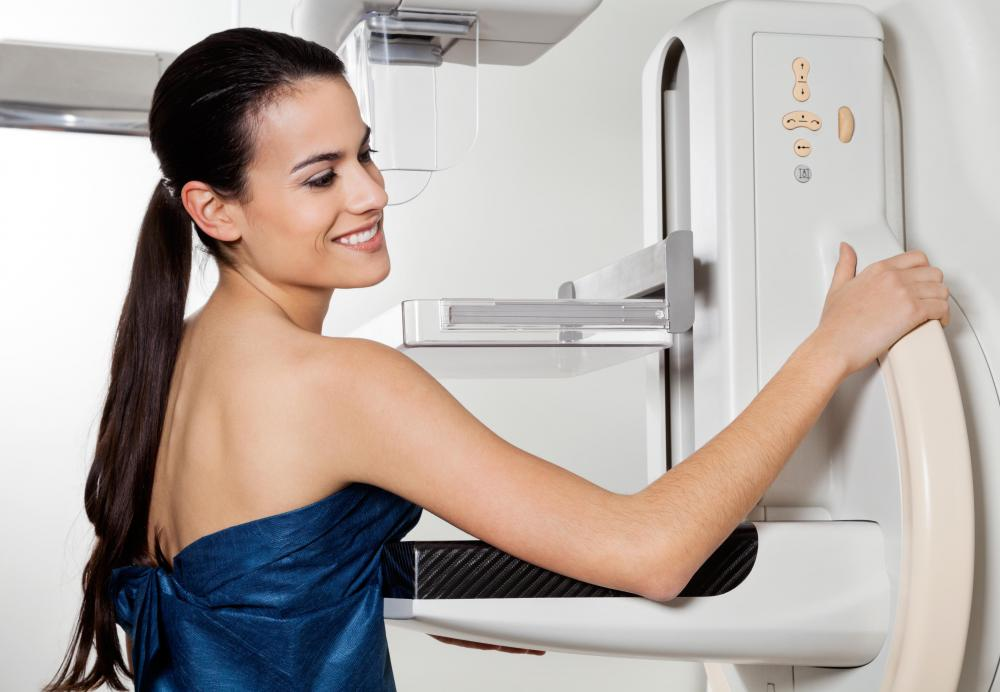# What is an Electrical Impedance? (with pictures)

Robert Robillard
Robert Robillard

Electrical impedance, often simply called impedance, is a measurement used to describe how easily electricity flows through an alternating current (AC) circuit. It describes the total amount of opposition that a circuit presents to the current. In a direct current (DC) circuit, resistance is used to describe the same properties, but in an AC system, additional factors come into play. Electrical impedance is a function of both resistance and reactance, because of the cyclical nature of an alternating current circuit.EIT may be used in conjunction with traditional medical imaging such as mammography to detect breast cancer.

Generally, the resistance of the circuit is determined by the number of free electrons available within the conductors to carry electrical current. A large diameter copper wire, for instance, will have more free electrons than a smaller diameter copper wire, and it will present a lower resistance. The type of metal used for the conductor also plays a role, because a less conductive metal has fewer free electrons and offers higher resistance to the current.

The second component of electrical impedance, reactance, is because of the cycling of an alternating electrical current. A typical household electrical current reverses direction 60 times per second, or at a rate of 60 Hertz. Each cycle causes additional electrical and magnetic opposition to the current. This opposition is called reactance.

Like resistance, the unit of measurement for electrical impedance is the ohm. Impedance is usually expressed as the difference in potential voltage across a circuit, divided by the maximum amount of current passing through the circuit. The simplest expression of electrical impedance is shown in the mathematical equation Z=V/I, in which Z represents impedance, V is voltage and I represents current.

Electrical measurements are useful for more than just copper wires and circuit boards. The human body also possesses a certain amount of conductivity, resistance and even impedance. In fact, the medical community has been using electrical impedance as a diagnostic tool since the early 1900s. Medical tests that rely on impedance take advantage of the fact that different types of tissue possess different magnitudes of impedance.

Electrical impedance imaging techniques, such as electrical impedance tomography (EIT), have been used for many years, and they have continued to improve and gain in popularity. Since malignant tissue typically shows lower impedance than healthy tissue, this type of testing has shown great promise in early detection of breast cancer. A typical EIT test involves placing electrodes on the body part being tested. A weak electrical current is applied to two or more electrodes, and the differences in voltage are measured. The test might be repeated several times and over many combinations of electrodes to gain the most accurate results.

## You might also Like